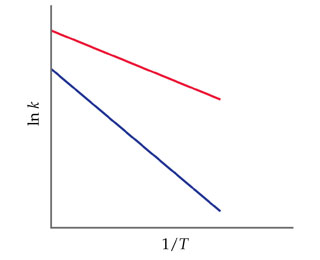# Problem: The accompanying graph shows plots of ln k versus 1/T for two different reactions. The plots have been extrapolated to the y-intercepts.Which reaction has the larger value for Ea?

###### FREE Expert Solution

In the problem, we are asked to find which of the two plots has a larger value for the activation energy (EA).

The Arrhenius Equation shows the dependence of the rate constant on the pre-exponential factor, absolute temperature and activation energy of the reaction

Mathematically, the Arrhenius Equation is expressed as:

$\overline{){\mathbf{k}}{\mathbf{=}}{{\mathbf{Ae}}}^{\mathbf{-}{\mathbf{E}}_{\mathbf{a}}}{\mathbf{RT}}}}$

Where:
k is the rate constant
A is the frequency factor
Ea is the activation energy
R is the gas constant (8.314 J/mol•K )
T is the temperature

To solve this problem we must relate the Arrhenius Equation to the plot of the graph.

97% (97 ratings)###### Problem Details

The accompanying graph shows plots of ln k versus 1/T for two different reactions. The plots have been extrapolated to the y-intercepts.Which reaction has the larger value for Ea?

Frequently Asked Questions

What scientific concept do you need to know in order to solve this problem?

Our tutors have indicated that to solve this problem you will need to apply the Arrhenius Equation concept. You can view video lessons to learn Arrhenius Equation. Or if you need more Arrhenius Equation practice, you can also practice Arrhenius Equation practice problems.

What professor is this problem relevant for?

Based on our data, we think this problem is relevant for Professor Zellmer's class at OSU.